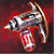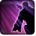# [speed_boost]Conditions

## Related effects, buffs and debuffs

• [5s]Speed Boost

 Slot: Buff Duration: 5s Tick rate: does not tick Initial stacks: 1 Max stacks: 1 # occurrences: 1
• When stacks of this effect increase
• When effect is applied

Perform the following actions:

• Set movement speed to 275%
• Custom
- Param 4 = (string)
- Param 5 = (string)
- Param 6 = (string)
- Param 7 = (string)
- Param 8 = (string)
- Param 9 = (string)
- Param 1 = (int) 9399188432865711031
- Param 3 = (int) 0
- Param 2 = (float) 75
• Just before this effect is removed

Perform the following actions:

• Custom
- Param 4 = (string)
- Param 5 = (string)
- Param 6 = (string)
- Param 7 = (string)
- Param 8 = (string)
- Param 9 = (string)
- Param 1 = (int) 9399188432865711031
- Param 3 = (int) 0
- Param 2 = (float) 70